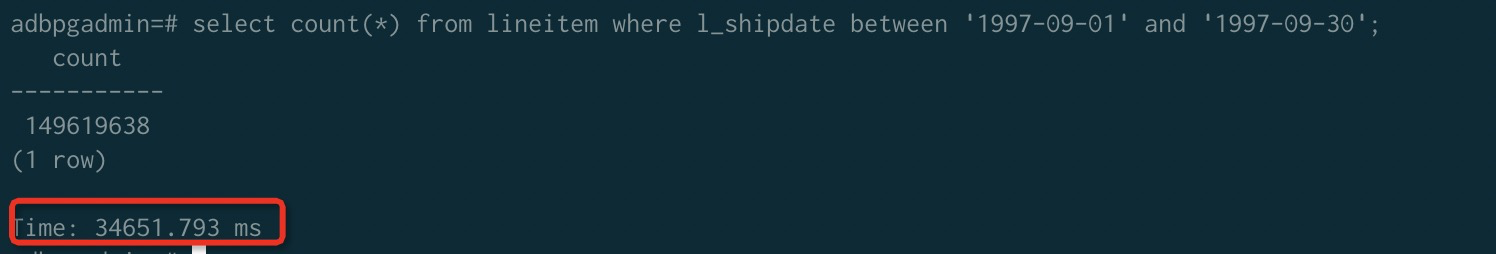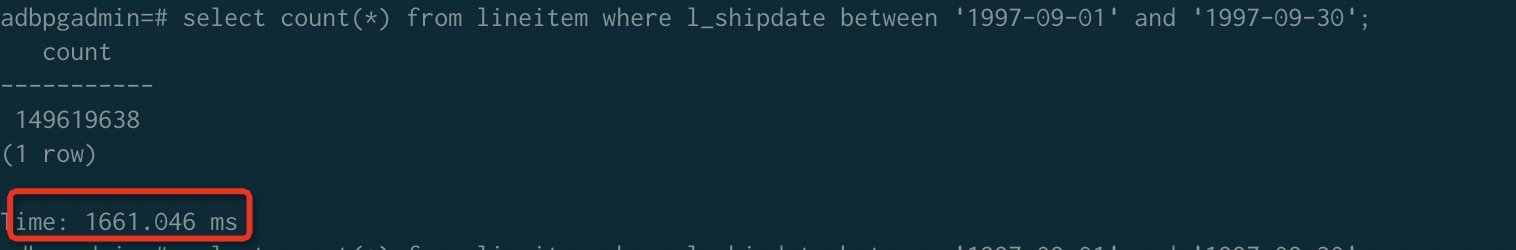This topic describes how to use sort keys and rough set indexes to improve query performance in column-oriented tables.

Notice This topic applies to the following instances:
• Newly created instances in reserved mode, whose kernel version is later than 20200826.
• Newly created instances in elastic mode, whose kernel version is later than 20200906.

## Background information

When you create a table, you can define one or more columns as the sort key. After data is written to the table, you can sort the table data by sort key.

Sorting accelerates range-restricted queries. The minimum and maximum values for each column are stored in a database. If a query restricts the range in the WHERE clause, the query processor of AnalyticDB for PostgreSQL can use the minimum and maximum values to skip blocks out of the specific range during table scans.

For example, assume that a table stores seven years of data sorted by date, and a query specifies a date range of one month. In this case, only 1/(7 × 12) of the table data needs to be scanned, and 98.8% of the disk blocks can be eliminated from the scan. If the data is not sorted by date, all disk blocks may be scanned.

AnalyticDB for PostgreSQL supports the following sorting methods:
• Compound sorting: applies to scenarios where the confining condition (constraint in the WHERE clause) is a prefix subset of the sort key or consists of all columns of the sort key. This sorting method is more useful for scenarios where the query condition includes the primary column of the confining condition.
• Interleaved sorting: gives an equal weight to each column in the sort key. This sorting method is more useful for scenarios where the query condition includes a subset of the confining condition.
For more information, see the "Performance comparison between compound sorting and interleaved sorting" section of this topic.
Performance comparison
This section provides an example on how compound sorting improves query performance for rough set indexes when compared with a full table scan.

A TPC-H Lineitem table that stores seven years of data is used in this example. Then, queries on data that uses and does not use the l_shipdate field are compared. Both queries are range-restricted.

Note This implementation of TPC is derived from the TPC Benchmark and is not comparable to published TPC Benchmark results, as this implementation does not comply with all the requirements of the TPC Benchmark.
Test procedure:
1. Create a 32-node instance.
2. Write 13 billion rows to the Lineitem table.
3. Query data in a time range from 1997-09-01 to 1997-09-30.
• Data is not sorted by l_shipdate.• Data is sorted by l_shipdate.## Define columns as the sort key when you create a table

Example

``create table test(date text, time text, open float, high float, low float, volume int) with(APPENDONLY=true,ORIENTATION=column) ORDER BY (volume);``

Syntax

``````CREATE [[GLOBAL | LOCAL] {TEMPORARY | TEMP}] TABLE table_name (
[ { column_name data_type  ...} ]
)
[ DISTRIBUTED BY (column, [ ... ] ) | DISTRIBUTED RANDOMLY ]
[ ORDER BY (column, [ ... ] )]``````

If the kernel version is earlier than 20210326, use the following statement to specify the sort key: SORTKEY (column, [ ... ])

## Sort a table

Use compound sorting to sort data
``SORT [tablename]``

If the kernel version is earlier than 20210326, use the following statement to specify the sort key:

``VACUUM SORT ONLY [tablename]``
Use interleaved sorting to sort data
``MULTISORT [tablename]``

If the kernel version is earlier than 20210326, use the following statement to specify the sort key:

``VACUUM REINDEX [tablename]``

After you execute the `SORT` or `MULTISORT` statement on a table, the table is sorted based on the specified sort key. As you add rows to the sorted table, the amount of unsorted data increases and filtering performance in rough sets may degrade. To ensure the filtering performance in rough sets, you must execute the `SORT` or `VACUUM REINDEX` (MULTISORT) statement to resort the table on a regular basis.

## Modify sort keys

You can execute the following statement to modify the sort key of an existing column-oriented table based on your business requirements:

``ALTER [[GLOBAL | LOCAL] {TEMPORARY | TEMP}] TABLE table_name SET ORDER BY (column, [ ... ] )``

This statement modifies only the catalog and does not immediately sort the data. To sort the data, you must execute the `SORT table_name` statement.

Example

``ALTER TABLE test SET ORDER BY(high,low);``

If the kernel version is earlier than 20210326, use the following statement to modify the sort key:

``ALTER TABLE test SET SORTKEY(high,low);``

## Specify a sort key and choose a sorting method

If you always need to run SQL queries that contain one or more column values or that are range-restricted, such as date columns, you can use those columns as sort keys. This accelerates the preceding SQL queries by sorting data based on rough set indexes.

We recommend that you use compound sorting in general cases. Compound sorting needs to perform extra analysis on the data. Therefore, `VACUUM REINDEX` can take longer than `VACUUM SORT ONLY` for interleaved tables.

If you seldom need to run SQL queries that contain specific columns, you can use interleaved sorting to accelerate queries. An interleaved sort key can use up to eight columns.

## Performance comparison between compound sorting and interleaved sorting

In this section, two tables that contain the same data are separately sorted by using compound sorting and interleaved sorting. The results of queries on the tables show that the two sorting methods show different performance levels in different scenarios.

Two tables with the same four columns (id, num1, num2, and value) are used in this example. (id,num1,num2) is specified as the sort key. Each table contains a total of 10 million rows. The tables used in this example are not particularly large tables for AnalyticDB for PostgreSQL, but the performance difference between compound sorting and interleaved sorting can be seen with the tables. The performance difference is more significant in large tables.

Test procedure:
1. Create two tables (test and test_multi) and set the same sort key for them.
2. Write test data to the tables.
3. Sort the test table by using compound sorting and sort the test_multi table by using interleaved sorting.
4. Compare the point query performance between compound sorting and interleaved sorting in the same SQL query.
5. Compare the range query performance between compound sorting and interleaved sorting in the same SQL query.
Create two tables and set the same sort key for them
``````CREATE TABLE test(id int, num1 int, num2 int, value varchar)
with(APPENDONLY=TRUE, ORIENTATION=column)
DISTRIBUTED BY(id)
ORDER BY(id, num1, num2);

CREATE TABLE test_multi(id int, num1 int, num2 int, value varchar)
with(APPENDONLY=TRUE, ORIENTATION=column)
DISTRIBUTED BY(id)
ORDER BY(id, num1, num2);``````
Write 10 million rows of data to each table
``````INSERT INTO test(id, num1, num2, value) select g,
(random()*10000000)::int,
(random()*10000000)::int,
(array['foo', 'bar', 'baz', 'quux', 'boy', 'girl', 'mouse', 'chlid', 'phone'])[floor(random() * 10 +1)]
FROM generate_series(1, 10000000) as g;

INSERT INTO test_multi SELECT * FROM test;

count
----------
10000000
(1 row)

count
----------
10000000
(1 row)``````
Separately sort the two tables by using compound sorting and interleaved sorting
``````SORT test;
MULTISORT test_multi;``````
Compare the point query performance
• The query is filtered on the primary column.
``````-- Q1 is filtered on the primary column.
select * from test where id = 100000;
select * from test_multi where id = 100000;``````
• The query is filtered on the second column.
``````-- Q2 is filtered on the second column.
select * from test where num1 = 8766963;
select * from test_multi where num1 = 8766963;``````
• The query is filtered on the second and third columns.
``````-- Q3 is filtered on the second and third columns.
select * from test where num1 = 100000 and num2=2904114;
select * from test_multi where num1 = 100000 and num2=2904114;``````
Table 1. Performance comparison results
Sorting method Q1 Q2 Q3
Compound sorting 0.026s 3.95s 4.21s
Interleaved sorting 0.55s 0.42s 0.071s
Compare the range query performance
• The query is filtered on the primary column.
``````-- Q1 is filtered on the primary column.
select count(*) from test where id>5000 and id < 100000;
select count(*) from test_multi where id>5000 and id < 100000;``````
• The query is filtered on the second column.
``````-- Q2 is filtered on the second column.
select count(*) from test where num1 >5000 and num1 <100000;
select count(*) from test_multi where num1 >5000 and num1 <100000;``````
• The query is filtered on the second and third columns.
``````-- Q3 is filtered on the second and third columns.
select count(*) from test where num1 >5000 and num1 <100000; and num2 < 100000;
select count(*) from test_multi where num1 >5000 and num1 <100000 and num2 < 100000;``````
Table 2. Performance comparison results
Sorting method Q1 Q2 Q3
Compound sorting 0.07s 3.35s 3.64s
Interleaved sorting 0.44s 0.28s 0.047s
Test conclusion
• Q1 uses the primary column of the sort key to filter data. In this case, compound sorting has a shorter query response time than interleaved sorting.
• Q2 uses a non-primary key column of the sort key to filter data. In this case, compound sorting is ineffective and interleaved sorting has significantly better query performance.
• Q3 uses non-primary key columns of the sort key to filter data. In this case, interleaved sorting is faster and more effective than compound sorting. The more columns of interleaved sort keys used, the better the performance of the interleaved sorting query.